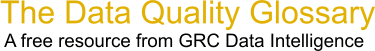Choose Index below for a list of all words and phrases defined in this glossary.

# Total Probability of Defect

index | Index

## Total Probability of Defect - definition(s)

Total Prob of Defect - The total probability of defect is equal to the sum of the probability of defect above the upper spec limit-p(d), upper-and the probability of defect below the lower spec limit-p(d), lower.

[Category=Data Quality ]

Source: iSixSigma, 28 February 2011 10:21:31, http://www.isixsigma.com/index.php?option=com_glossaryData Quality Glossary.  A free resource from GRC Data Intelligence. For comments, questions or feedback: dqglossary@grcdi.nl